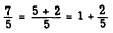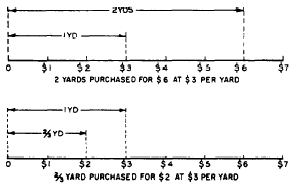Chapter 4 - COMMON FRACTIONSCustom SearchCHAPTER 4 COMMON FRACTIONS The emphasis in previous chapters of this course has been on integers (whole numbers). In this chapter, we turn our attention to numbers which are not integers. The simplest type of number other than an integer is a COMMON FRACTION. Common fractions and integer8 together comprise a set of numbers called the RATIONAL NUMBERS; this set is a subset of the set of real numbers. The number line may be used to show the relationship between integers and fractions. For example, if the interval between 0 and 1 is marked off to form three equal spaces (thirds), then each space so formed is one-third of the total interval. If we move along the number line from 0 toward 1, we will have covered two of the three "thirds" when we reach the second mark. Thus the position of the second mark represents the number 2/3. (See fig. 4-l.)Figure 4-l.-Integers and fractions on the number line. The numerals 2 and 3 in the fraction 2/3 are named so that we may distinguish between them; 2 is the NUMERATOR and 3 is the DENOMINATOR. In general, the numeral above the dividing line in a fraction is the numerator and the numeral below the line is the denominator. The numerator and denominator are the TERMS of the fraction. The word "numerator" is related to the word "enumerate." To enumerate means to "tell how many"; thus the numerator tells us how many fractional parts we have in the indicated fraction. To denominate means to "give a name" or "tell what kind"; thus the denominator tells us what kind of parts we have (halves, thirds, fourths, etc.). Attempts to define the word "fraction" in mathematics usually result in a statement similar to the following: A fraction is an indicated division. Any division may be indicated by placing the dividend over the divisor and drawing a line between them. By this definition, any number which can be written as the ratio of two integers (one integer over the other) can be considered as a fraction. This leads to a further definition: Any number which can be expressed as the ratio of two integers is a RATIONAL number. Notice that every integer is a rational number, because we can write any integer as the numerator of a fraction having 1 as its denominator. For example, 5 is the same as 5/1. It should be obvious from the definition that every common fraction is also a rational number. TYPES OF FRACTIONS Fractions are often classified as proper or improper. A proper fraction is one in which the numerator is numerically smaller than the denominator. An improper fraction has a numerator which is larger than its denominator. MIXED NUMBERS  When the denominator of an improper fraction is divided into its numerator, a remainder is produced along with the quotient, unless the numerator happens to be an exact multiple of the denominator. For example, 7/5 is equal to 1 plus a remainder of 2. This remainder may be shown as a dividend with 5 as its divisor, as follows:The expression 1 t 2/5 is a MIXED NUMBER. Mixed numbers are usually written without showing the plus sign; that is, 1 + 2/5 is the same as 1 2/5 or 1 2/5. When a mixed number is written as 1 2/5, care must be taken to insure that there is a space between the 1 and the 2; otherwise, 1 2/5 might be taken to mean 12/5. MEASUREMENT FRACTIONS Measurement fractions occur in problems such as the following: If \$2 were spent for a stateroom rug at \$3 per yard, how many yards were bought? If \$6 had been spent we could find the number of yards by simply dividing the cost per yard into the amount spent. Since 6/3 is 2, two yards could be bought for \$6. The same reasoning applies when \$2 are spent, but in this case we can only indicate the amount purchased as the indicated division 2/3. Figure 4-2 shows a diagram for both the \$6 purchase and the \$2 purchase.Figure 4-2.-Measurement fractions. PARTITIVE FRACTIONS The difference between measurement fractions and partitive fractions is explained as follows: Measurement fractions result when we determine how many pieces of a given size can be cut from a larger piece. Partitive fractions result when we cut a number of pieces of equal size from a larger piece and then determine the size of each smaller piece. For example, if 4 equal lengths of pipe are to be cut from a S-foot pipe, what is the size of each piece? If the problem had read that 3 equal lengths were to be cut from a 6-foot pipe, we could find the size of each pipe by dividing the number of equal lengths into the overall length. Thus, since 6/3 is 2, each piece would be 2 feet long. By this same reasoning in the example, we divide the overall length by the number of equal parts to get the size of the individual pieces; that is, 3/4 foot. The partitioned 6-foot and J-foot pipes are shown in figure 4-3.Figure 4-3.-Partitive fractions EXPRESSING RELATIONSHIPS When a fraction is used to express a relationship, the numerator and denominator take on individual significance. In this frame of reference, 3/4 means 3 out of 4, or 3 parts in 4, or the ratio of 3 to 4. For example, if 1 out of 3 of the men in a division are on liberty, then it would be correct to state that l/3 of the division are on liberty. Observe that neither of these ways of expressing the relationship tells us the actual number of men; the relationship itself is the important thing, Practice problems. 1. What fraction of 1 foot is 11 inches? 2. Represent 3 out of 8 as a fraction. 3. Write the fractions that indicate the relationship of 2 to 3; 8 divided by 9; and 6 out of 7 equal parts 4.Answers: 1. 11/12 2. 3/8 3. 2/3; 8/9; 6/7 4. plusIntegrated Publishing, Inc. - A (SDVOSB) Service Disabled Veteran Owned Small Business